# Parameter Estimation of Rayleigh-Generalized Gamma Mixture Model

Parameter Estimation of Rayleigh-Generalized Gamma Mixture Model
Département d’Electronique, Université Mohamed Boudiaf-Msila, Msila 28000, Algérie

Laboratoire Signaux et Systèmes de Communications (SISCOM), Université de Constantine, Constantine 25000, Algérie

Laboratoire LGE, Université Mohamed Boudiaf- Msila, Msila 28000, Algérie

Corresponding Author Email:
amar.mezache@univ-msila.dz
Page:
59-64
|
DOI:
https://doi.org/10.18280/i2m.190108
20 October 2019
|
Accepted:
2 December 2019
|
Published:
20 March 2020
| Citation

OPEN ACCESS

Abstract:

The estimation problem of three parameters Rayleigh-Generalized Gamma Mixture (R-GG) radar clutter model is addressed in this paper. Expressions of integer order moments, non-integer order moments and logarithmic moments are presented in such away the scale parameter of the R-GG probability density function (PDF) is eliminated and a two-dimensional estimators labeled HOME, NIOME and [zlog(z)] methods are obtained. Due to the presence of gamma function with fractional variables, these estimators cannot be given in closed forms. The fitness function for each estimator is given as a sum of squared errors of nonlinear equations. Using a numerical routine based upon the simplex search algorithm, the proposed methods were tested firstly on artificial data. Tail fitting of the R-GG model and the standard K-distribution (i.e., special case of the R-GG) is assessed against recorded radar data. The accuracy of the R-GG model and the proposed estimation methods is satisfactory, with the most accuracy of the [zlog(z)] method.

Keywords:

radar clutter, parameter estimation, compound-gaussian, generalized gamma, HOME, NIOME, [zlog(z)]

1. Introduction

Radar clutter is defined as the unwanted reflective waves from irrelevant targets. Modeling of high resolution radar echoes is a key problem of target detection processors. Due to the phenomenon complexity of sea surface clutter, numerous clutter models have been proposed in the open literature [1-6]. The amplitude statistics of clutter have been firstly modeled by Rayleigh, log-normal, Weibull, K and mixture distributions. During the past thirty years there has been a considerable growth of interest in various texture components of compound-Gaussian distributed sea clutter returns. The study of statistical properties of sea clutter echoes is very complex. They not only depend on the type of background, but also on the parameters of the radar itself, such as resolution, wavelength, polarization, etc. . In addition, since the environment will change with time, the clutter signal also exhibits non-stationary statistics. This adds more complexity to the study of statistical properties of sea clutter. For this reason, it not possible to obtain a model which characterizes the statistics of sea or land clutter in all cases. Generally, previous works considered compound Gaussian models with two parameters of the texture components. Thus, we have to search always for alternative models for radar clutter [2-6]. For the general case, generalized compound (GC) clutter is proposed by Anastassopoulos et al. . Here, the speckle and the texture components are both fluctuated according to the generalized gamma law with different shape and power parameters. The popular K-distribution given by Bocquet  can be easily obtained from a particular case of the GC model parameters. It characterizes various types of radar backscattering and is considered by many researchers in radar signal processing schemes like especially in target detection with constant false alarm (CFAR) behavior. The K clutter model is one of the compound Gaussian (CG) distributions proposed in the open literature, but with a gamma law for the texture component. In order to achieve a goodness-of-fit to real life data, the modulation component for the CG model has been changed to be an inverse gamma law, an inverse Gaussian law, a log-normal law, etc. . The mixtures of two or more models are also accurate for the interference distribution . In this context, Abraham et al.  proposed a mixture of a Rayleigh and K distributions for representing active sonar data comprising clutter sparsely observed in a Rayleigh-distributed background. The K-Rayleigh mixture model was seen to provide improved PDF fits and inference on the clutter statistics. A parameter estimation technique based on the expectation maximization (EM) algorithm is proposed and shown to perform adequately. In order to give more properties of the mean clutter power (i.e., texture component), generalized gamma law with three parameters is considered by Anastassopoulos .

Parameter estimation of radar clutter is a very important task in modern radar technology. Concerning false alarms and effective detection processes, adaptive threshold requires the model parameters values estimated from the clutter range profile (CRP) . There are many papers discussing this problem [7-10]. Since the statistical models of clutter for different environments are different, the methods of parameter estimation will be also different for different types of clutter. For this reason, several techniques have been proposed based on least squares (curve fitting), matching of moments (HOME), maximum likelihood (MLE), etc. Integer order moments approach is commonly used for parameter estimation of numerous clutter models, but it provides suboptimal results . In the case of K clutter, it was shown that non integer moments and log moments can be manipulated to get closed form estimators labeled FOME and [zlog(z)] methods respectively [8, 9]. If the texture component is modeled by a log-normal law, closed forms of [zlog(z)] and NIOME methods are also derived recently  and yield a good estimation accuracy relative to the HOME method.

The purpose of this work is to derive three estimation approaches of the parameters of the R-GG radar clutter model. Recall that, this distribution has already been considered by Anastassopoulos , but the estimation methods based on the curve fitting and matching of moments were used. In this paper, we used different 2D estimation procedures in which a mixture of integer order moments, non-integer order moments and log moments are used. Corresponding formulas of these expressions of moments are presented in such away the scale parameter of the R-GG model is eliminated and a two-dimensional estimators labeled HOME, NIOME and [zlog(z)] methods are obtained. Due to the presence of gamma function with fractional variables, these estimators cannot be obtained in closed forms. The objective function for each estimator is given as a sum of squared errors of nonlinear equations. The simplex Nelder-Mead (N-M) search algorithm is used for optimization of the proposed estimation methods using artificial data. To show the efficiency of the proposed [zlog(z)] estimation procedure, tail fitting of the R-GG model and the standard K distribution (i.e., special case of the R-GG) are compared against recorded IPIX radar data.

The rest of the paper is outlined as follows. Section 2 presents the R-GG radar clutter model, its expression of moments and corresponding distribution function. Then, Section 3 gives manipulations of integer order moments, non-integer order moments and log moments in such away the estimation complexity of R-GG clutter parameters is reduced in two-dimensions. Section 4 evokes the minimization of three fitness functions by the N-M tool where the proposed estimators are assessed on both simulated and measured data. Finally, some conclusions are drawn in Section 5.

2. R-GΓ Clutter Distribution

In radar detection applications, several statistical models have been proposed to fit sea reverberation data [1-6]. Appropriate model can have a significant impact on the success of detecting targets embedded within the sea surface. As discussed earlier, the GC model is obtained when both speckle and texture components follow generalized Gamma PDFs given by Sekine and Mao .

\left\{ \begin{align} & p\left( \left. x \right|y \right)=\frac{{{b}_{1}}}{y\Gamma \left( {{\nu }_{1}} \right)}{{\left( \frac{x}{y} \right)}^{{{b}_{1}}{{\nu }_{1}}-1}}\exp \left( -{{\left( \frac{x}{y} \right)}^{{{b}_{1}}}} \right) \\ & p\left( y \right)=\frac{{{b}_{2}}}{a\Gamma \left( {{\nu }_{2}} \right)}{{\left( \frac{y}{a} \right)}^{{{b}_{2}}{{\nu }_{2}}-1}}\exp \left( -{{\left( \frac{y}{a} \right)}^{{{b}_{2}}}} \right) \\ \end{align} \right.   (1)

where, a is the scale parameter, b1 and b2 are the power parameters, v1 and v2 are the shape parameters. The CG clutter model with generalized gamma texture law is obtained from (1) if b1=2 and v1=1. Hence, (1) becomes

\left\{ \begin{align} & p\left( \left. x \right|y \right)=\frac{2x}{{{y}^{2}}}\exp \left( -\frac{{{x}^{2}}}{{{y}^{2}}} \right) \\ & p\left( y \right)=\frac{{{b}_{2}}}{a\Gamma \left( {{\nu }_{2}} \right)}{{\left( \frac{y}{a} \right)}^{{{b}_{2}}{{\nu }_{2}}-1}}\exp \left( -{{\left( \frac{y}{a} \right)}^{{{b}_{2}}}} \right) \\ \end{align} \right.  (2)

Taking b2=b and v2=v, the overall PDF of (2) is

\begin{align} & {{p}_{X}}\left( x;a,b,\nu \right)=\int\limits_{0}^{+\infty }{p\left( \left. x \right|y \right)p\left( y \right)dy} \\ & \text{ }=\frac{2b}{\Gamma \left( \nu \right)}\frac{x}{{{a}^{b\nu }}}\int\limits_{0}^{+\infty }{{{y}^{b\nu -3}}\exp \left( -{{\left( \frac{y}{a} \right)}^{b}}-{{\left( \frac{x}{y} \right)}^{2}} \right)dy} \\\end{align}  (3)

Under the assumption of independent and identically distributed (iid) samples of the CRP, the expression of moments of order k is given by

\begin{align} & \left\langle {{x}^{k}} \right\rangle ={{\mu }_{k}}=\int\limits_{0}^{+\infty }{{{x}^{k}}p\left( x \right)dx} \\ & \text{ }={{a}^{k}}\frac{\Gamma \left( k/2+1 \right)\Gamma \left( k/b+\nu \right)}{\Gamma \left( \nu \right)} \\ \end{align}  (4)

where, $\langle\cdot\rangle$ denotes expectation. The clutter power is $\mu_{2}=a^{2} \Gamma(2 / b+v) / \Gamma(v)$. From (3), the complementary distribution function (CCDF) is given by

\begin{align} & CCDF\left( T;a,b,\nu \right)=\int\limits_{T}^{+\infty }{p\left( x \right)dx} \\ & \text{ }=\frac{b}{a\Gamma \left( \nu \right)}\int\limits_{0}^{+\infty }{{{\left( \frac{y}{a} \right)}^{b\nu -1}}\exp \left( -{{\left( \frac{y}{a} \right)}^{b}}-{{\left( \frac{T}{y} \right)}^{2}} \right)dy} \\\end{align}  (5)

where, T is the normalized detection threshold. Some artificial scenarios of the R-GG PDF are depicted in Figure 1 for b=1 and several values of v. Here, it is shown that the Rayleigh clutter case is obtained when v is large.

## 1.jpg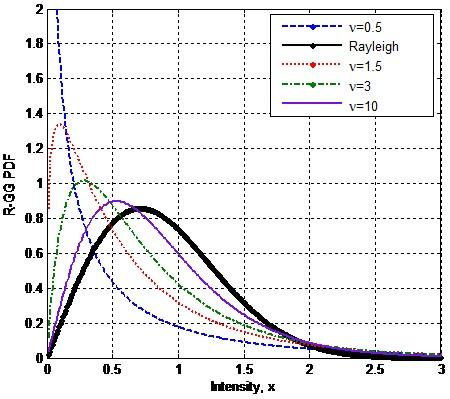Figure 1. Plots of the R-GG distribution given by (3) for b=1 and E[x2]=1 (i.e., $a=\sqrt{\Gamma \left( \nu \right)/\Gamma \left( 2/b+\nu \right)}$ )

The K-distribution is a special case of the R-G$\Gamma$  distribution. This is obtained when b=2 for which (3) is reformulated by

\begin{align} & {{p}_{X}}\left( x;a,\nu \right)\text{ }=\frac{4}{\Gamma \left( \nu \right)}\frac{x}{{{a}^{2\nu }}}\int\limits_{0}^{+\infty }{{{y}^{2\nu -3}}\exp \left( -{{\left( \frac{y}{a} \right)}^{2}}-{{\left( \frac{x}{y} \right)}^{2}} \right)dy} \\ & \text{ }=\frac{4{{a}^{-\nu -1}}{{x}^{\nu }}}{\Gamma \left( \nu \right)}{{K}_{\nu -1}}\left( 2\frac{x}{a} \right) \\\end{align}  (6)

where, K(.) is the modified Bessel function of the second kind of order v-1 . The corresponding CCDF of (6) will be

$CCDF\left( T;a,\nu \right)=\frac{2{{a}^{-\nu }}}{\Gamma \left( \nu \right)}{{T}^{\nu }}{{K}_{\nu }}\left( 2\frac{T}{a} \right)$  (7)

3. Estimators

In the following, underling expressions are developed under the condition of iid samples where x1, x2, …, xM represent M independent magnitudes of radar clutter. From (4), combination of moments of orders 3 and 4 denoted by m3 and m4 yields the following formula.

$\frac{{{\mu }_{3}}\Gamma {{\left( 3 \right)}^{3/4}}}{\mu _{4}^{3/4}\Gamma \left( 5/2 \right)}=\frac{\Gamma \left( 3/b+\nu \right)\Gamma {{\left( \nu \right)}^{-1/4}}}{\Gamma {{\left( 4/b+\nu \right)}^{3/4}}}$   (8)

On the other hand, the manipulation of the first two integer moments, m1 and m2 gives

$\frac{{{\mu }_{1}}}{\sqrt{{{\mu }_{2}}}}=\frac{\Gamma \left( 1.5 \right)\Gamma \left( 1/b+\nu \right)}{\sqrt{\Gamma \left( \nu \right)\Gamma \left( 2/b+\nu \right)}}$  (9)

If we combine the first order moment, m1 with non-integer moments, mk and mk+1, the following expression as a function of b and v is obtained

$\frac{{{\mu }_{k+1}}\Gamma \left( 3/2 \right)\Gamma \left( k/2+1 \right)}{\mu {{\mu }_{k}}\Gamma \left( \left( k+1 \right)/2+1 \right)}=\frac{\Gamma \left( \nu \right)\Gamma \left( \left( k+1 \right)/b+\nu \right)}{\Gamma \left( 1/b+\nu \right)\Gamma \left( k/b+\nu \right)}$  (10)

It is observed that the scale parameter a is eliminated in (8)-(10). Log moments can also be used to obtain modified expression without a. To do this, we take firstly the derivative of (4) with respect to k. Hence

\begin{align} & \frac{\partial \left\langle {{x}^{k}} \right\rangle }{\partial k}=\left\langle {{x}^{k}}\log \left( x \right) \right\rangle \\ & \text{ }=\frac{{{a}^{k}}\log \left( a \right)\Gamma \left( k/2+1 \right)\Gamma \left( k/b+\nu \right)}{\Gamma \left( \nu \right)} \\ & \text{ }+\frac{\frac{1}{2}{{a}^{k}}\Gamma \left( k/2+1 \right)\psi \left( k/2+1 \right)\Gamma \left( k/b+\nu \right)}{\Gamma \left( \nu \right)} \\ & \text{ }+\frac{\frac{1}{b}{{a}^{k}}\Gamma \left( k/2+1 \right)\Gamma \left( k/b+\nu \right)\psi \left( k/b+\nu \right)}{\Gamma \left( \nu \right)} \\\end{align}  (11)

For k=0, (11) is simplified to

$\left\langle \log \left( x \right) \right\rangle =\log \left( a \right)+\frac{1}{2}\psi \left( 1 \right)+\frac{1}{b}\psi \left( \nu \right)$  (12)

For k=1, (11) becomes

\begin{align} & \left\langle x\log \left( x \right) \right\rangle =\frac{a\Gamma \left( 1/2+1 \right)\Gamma \left( 1/b+\nu \right)}{\Gamma \left( \nu \right)} \\ & \text{ }\left[ \log \left( a \right)+\frac{1}{2}\psi \left( 1/2+1 \right)+\frac{1}{b}\psi \left( 1/b+\nu \right) \right] \\\end{align}  (13)

The combination of $\mu_{I}=\langle x\rangle$ with the above $\langle\log (x)\rangle$ and $\langle x\log (x)\rangle$ formulas yields.

$\frac{\left\langle x\log \left( x \right) \right\rangle }{\left\langle x \right\rangle }-\left\langle \log \left( x \right) \right\rangle -1+\log 2=\frac{1}{b}\left( \psi \left( 1/b+\nu \right)-\psi \left( \nu \right) \right)$  (14)

where, $\frac{1}{2}\left( \psi \left( 1/2 \right)-\psi \left( 1 \right) \right)=-\log 2$ is used .

Note that, $\mu_{I}=\langle x\rangle$ which means the first order moment is combined by (12) and (13) to yield the [zlog(z)] based estimator in terms of $\widehat{b}$ and $\widehat{v}$ as given by (14). This combination was used to reduce the number of variables of such estimation procedure.

If b=2 which means the K-clutter case, closed form [zlog(z)] estimator is obtained when k=2 in (11)

$\hat{\nu }={{\left[ 2\left( \frac{\left\langle {{x}^{2}}\log \left( x \right) \right\rangle }{\left\langle {{x}^{2}} \right\rangle }-\left\langle \log \left( x \right) \right\rangle \right)-1 \right]}^{-1}}$  (15)

Note that the scale parameter, a is not appeared in (8), (9), (10) and (14). This two-dimensional estimation problem can be solved by the solution of system with two nonlinear equations. In the next subsections, it will be shown that three nonlinear systems from (8), (9), (10) and (14) can be obtained.

3.1 Higher order moments based estimator

This method is referred to as HOME where (8) and (9) are used for the solution of the following system.

\left\{ \begin{align} & \frac{{{\mu }_{3}}\Gamma {{\left( 3 \right)}^{3/4}}}{\mu _{4}^{3/4}\Gamma \left( 5/2 \right)}-\frac{\Gamma \left( 3/\hat{b}+\hat{\nu } \right)\Gamma {{\left( {\hat{\nu }} \right)}^{-1/4}}}{\Gamma {{\left( 4/\hat{b}+\hat{\nu } \right)}^{3/4}}}=0 \\ & \frac{{{{\hat{\mu }}}_{1}}}{\sqrt{{{{\hat{\mu }}}_{2}}}}-\frac{\Gamma \left( 1.5 \right)\Gamma \left( 1/\hat{b}+\hat{\nu } \right)}{\sqrt{\Gamma \left( {\hat{\nu }} \right)\Gamma \left( 2/\hat{b}+\hat{\nu } \right)}}=0 \\\end{align} \right.  (16)

3.2 Non-integer order moments based estimator

This estimator is referred to as NIOME method where (9) and (10) are handled

\left\{ \begin{align} & \frac{{{\mu }_{k+1}}\Gamma \left( 3/2 \right)\Gamma \left( k/2+1 \right)}{\mu {{\mu }_{k}}\Gamma \left( \left( k+1 \right)/2+1 \right)}-\frac{\Gamma \left( {\hat{\nu }} \right)\Gamma \left( \left( k+1 \right)/\hat{b}+\hat{\nu } \right)}{\Gamma \left( 1/\hat{b}+\hat{\nu } \right)\Gamma \left( k/\hat{b}+\hat{\nu } \right)}=0 \\ & \frac{{{{\hat{\mu }}}_{1}}}{\sqrt{{{{\hat{\mu }}}_{2}}}}-\frac{\Gamma \left( 1.5 \right)\Gamma \left( 1/\hat{b}+\hat{\nu } \right)}{\sqrt{\Gamma \left( {\hat{\nu }} \right)\Gamma \left( 2/\hat{b}+\hat{\nu } \right)}}=0 \\\end{align} \right. (17)

3.3 Log moments based estimator

That estimator is referred to as [zlog(z)] method where (9) and (14) are considered for solving the system below.

\left\{ \begin{align} & A+\log \left( 2 \right)-\frac{1}{b}\left( \psi \left( 1/\hat{b}+\hat{\nu } \right)-\psi \left( {\hat{\nu }} \right) \right)=0 \\ & \frac{{{{\hat{\mu }}}_{1}}}{\sqrt{{{{\hat{\mu }}}_{2}}}}-\frac{\Gamma \left( 1.5 \right)\Gamma \left( 1/\hat{b}+\hat{\nu } \right)}{\sqrt{\Gamma \left( {\hat{\nu }} \right)\Gamma \left( 2/\hat{b}+\hat{\nu } \right)}}=0 \\\end{align} \right.  (18)

where, $A=\frac{\left\langle z\log \left( z \right) \right\rangle }{\left\langle z \right\rangle }-\left\langle \log \left( z \right) \right\rangle -1$.

After computing b and v from (16), (17) or (18), the parameter a is simply computed using the first integer moment. Hence

$\text{ \hat{a}}=\frac{\Gamma \left( \nu \right){{{\hat{\mu }}}_{\text{1}}}}{\Gamma \left[ 3/2 \right]\Gamma \left( 1/\hat{b}+\hat{\nu } \right)}$  (19)

where, $\text{ }{{\hat{\mu }}_{\text{k}}}=\frac{1}{N}\sum\limits_{i=1}^{N}{{{({{x}_{i}})}^{k}}}$ is calculated from the data.

4. Estimation Analysis

It is now of interest to compare the performance of the new estimators given by (16)-(19). This will be done by generating artificial R-GG distributed clutter, with discrete shape parameter values between 0.1 and 1.5. For some values of b = 0.5, 1, 2 and 3, the clutter power is normalized to one so that $a=\sqrt{\Gamma \left( \nu \right)/\Gamma \left( 2/b+\nu \right)}$.

These values have been selected to cover very spiky and spiky clutter situations. To solve systems of nonlinear equations evoked in Section 3, numerical routines are required. To this end, the fitness function is given in terms of the sum of squared errors for each equation. For the case of HOME method, we can write

\begin{align} & Fitness\left( \hat{b};\hat{\nu } \right)={{\left[ \frac{{{{\hat{\mu }}}_{3}}\Gamma {{\left( 3 \right)}^{3/4}}}{\hat{\mu }_{4}^{3/4}\Gamma \left( 5/2 \right)}-\frac{\Gamma \left( 3/\hat{b}+\hat{\nu } \right)\Gamma {{\left( {\hat{\nu }} \right)}^{-1/4}}}{\Gamma {{\left( 4/\hat{b}+\hat{\nu } \right)}^{3/4}}} \right]}^{2}} \\ & \text{ }+{{\left[ \frac{{{{\hat{\mu }}}_{1}}}{\sqrt{{{{\hat{\mu }}}_{2}}}}-\frac{\Gamma \left( 1.5 \right)\Gamma \left( 1/\hat{b}+\hat{\nu } \right)}{\sqrt{\Gamma \left( {\hat{\nu }} \right)\Gamma \left( 2/\hat{b}+\hat{\nu } \right)}} \right]}^{2}} \\\end{align}  (20)

Similarly, the belief function of the NIOME method is

\begin{align} & Fitness\left( \hat{b};\hat{\nu } \right)=\left[ \frac{{{{\hat{\mu }}}_{k+1}}\Gamma \left( 3/2 \right)\Gamma \left( k/2+1 \right)}{\hat{\mu }{{{\hat{\mu }}}_{k}}\Gamma \left( \left( k+1 \right)/2+1 \right)} \right. \\ & \text{ }{{\left. \text{ -}\frac{\Gamma \left( {\hat{\nu }} \right)\Gamma \left( \left( k+1 \right)/\hat{b}+\hat{\nu } \right)}{\Gamma \left( 1/\hat{b}+\hat{\nu } \right)\Gamma \left( k/\hat{b}+\hat{\nu } \right)} \right]}^{\text{2}}}\text{ } \\ & \text{ }+{{\left[ \frac{{{{\hat{\mu }}}_{1}}}{\sqrt{{{{\hat{\mu }}}_{2}}}}-\frac{\Gamma \left( 1.5 \right)\Gamma \left( 1/\hat{b}+\hat{\nu } \right)}{\sqrt{\Gamma \left( {\hat{\nu }} \right)\Gamma \left( 2/\hat{b}+\hat{\nu } \right)}} \right]}^{2}} \\\end{align}  (21)

Finally, the belief function for the case of mixture [zlog(z)] and the first two integer moments based methods yields

\begin{align} & Fitness\left( \hat{b};\hat{\nu } \right)={{\left[ \hat{A}+\log \left( 2 \right)-\frac{1}{b}\left( \psi \left( 1/\hat{b}+\hat{\nu } \right)-\psi \left( {\hat{\nu }} \right) \right) \right]}^{2}} \\ & \text{ }+{{\left[ \frac{{{{\hat{\mu }}}_{1}}}{\sqrt{{{{\hat{\mu }}}_{2}}}}-\frac{\Gamma \left( 1.5 \right)\Gamma \left( 1/\hat{b}+\hat{\nu } \right)}{\sqrt{\Gamma \left( {\hat{\nu }} \right)\Gamma \left( 2/\hat{b}+\hat{\nu } \right)}} \right]}^{2}} \\\end{align}  (22)

To solve (20)-(22), we used the Matlab function “fminsearch” to find the minimum with two variables, starting at an initial estimate. This is generally referred to as unconstrained nonlinear optimization. The “fminsearch” uses the simplex search method of [12 and therein]. This is a direct search method that does not use numerical or analytic gradients. The Nelder-Mead simplex algorithm uses a simplex of n+1 points for n-dimensional vectors x. The algorithm first makes a simplex around the initial guess x0 by adding 5% of each component x0(i) to x0, and using these n vectors as elements of the simplex in addition to x0. It uses 0.00025 as component i if x0(i) = 0.

4.1 Estimation using simulated data

## 2.pngFigure 2. MSE and Bias estimates using HOME, NIOME and [zlog(z)] based methods for b=0.5 and M=10000

The following Matlab function is used to get R-GG distributed M independent samples.

\left\{ \begin{align} & y=a{{\left[ \text{gammaincinv}\left( \text{rand}\left( M\text{,1} \right)\text{,}\nu \right) \right]}^{\frac{1}{b}}} \\ & \text{x}=\text{raylrnd}\left( \frac{y}{\sqrt{\text{2}}} \right) \\\end{align} \right.  (23)

where, $\text{gammaincinv}\left( \text{.,}\text{.} \right)$ and $\text{raylrnd}\left( . \right)$ are the inverse incomplete gamma and Rayleigh functions respectively. For consistency, the CRP size M is set to 10000 throughout. Monte-Carlo simulation is used to estimate the bias ($Bias=\left[ \left| \nu -\hat{\nu } \right| \right]$) and the mean square errors ($MSE=\left[ {{\left( \nu -\hat{\nu } \right)}^{2}} \right]$) using n=100 runs. The fractional order is set to k=0.1 in all simulations. Figure 2 shows estimation performance as a function of v in the case of a small value of the power parameter b=0.5. It is clear, that the [zlog(z)] based estimator exhibits the best performance. Figure 3 repeats the same experiment except with b=1. The NIOME method provides almost the same results relative to the [zlog(z)] approach. The HOME method offers improved MSE and bias values when v tends to 0.2. If the clutter is considered as a K-clutter with b=2, Figure 4 depicts values of MSE and bias in terms of v. Also, similar performance of NIOME and [zlog(z)] is obtained. We can observe that there is a week interval of v in which the HOME gives the better estimates. If the b is large (b=3), Figure 5 illustrates performance comparison of the proposed estimators. It is noticed that the HOME method is the best for 0.5<v<0.9. Comparing Figures 2-5, one concludes that estimator (22) performs almost ideally, due to the use of logarithmic moments. However, as b increases, it is clearly seen that the NIOME and [zlog(z)] methods perform comparably with HOME method. Also, these figures show that when the power parameter is increased more significantly, NIOME and [zlog(z)] procedures behave very similarly.

## 3.png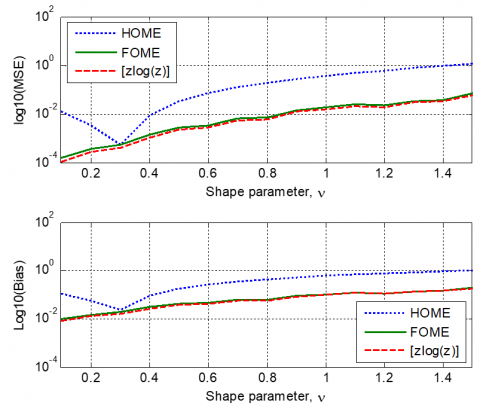Figure 3. MSE and Bias estimates using HOME, NIOME and [zlog(z)] based methods for b=1 and M=10000

## 4.png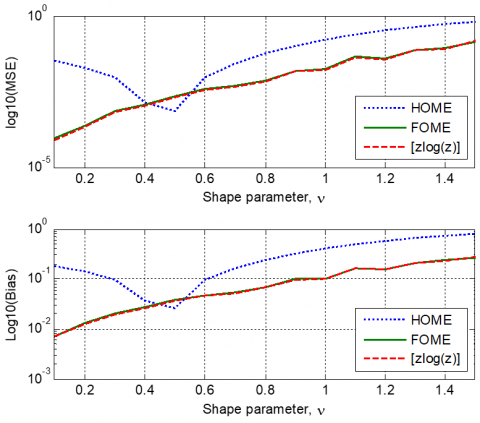Figure 4. MSE and Bias estimates using HOME, NIOME and [zlog(z)] based methods for b=2 (K-clutter case) and M=10000

## 5.pngFigure 5. MSE and Bias estimates using HOME, NIOME and [zlog(z)] based methods for b=3 and M=10000

4.2 Estimation using real data

In this subsection, the new estimator given by (22) is used to fit the R-GG model to IPIX real data. For comparison purposes, the K distribution is considered using the [zlog(z)] estimator given by (15). The lake-clutter data we processed were collected at Grimsby, Ontario, with the McMaster University IPIX radar. IPIX is experimental X-band search radar, capable of dual polarized and frequency agile operation . We focus our analysis on the datasets 84, 85 and 86 which correspond to the range resolutions 30m, 15m and 3m respectively. Characteristic features of the IPIX radar are found by Bakker and Currie . The radar site was located at east of the “Place Polonaise” at Grimsby, Ontario (Latitude 43: 2114±N, Longitude 79:5985±W), looking at lake Ontario from a height of 20 meter (m). The nearest shore on the far side of the lake is more than 20 Km away. The data of the Grimsby database are stored in 222 files, as 10 bits integers.

## 6.pngFigure 6. Fitting the R-GG and K-distributions to empirical data with HH polarization, resolution 3m and 13th range cell

## 7.png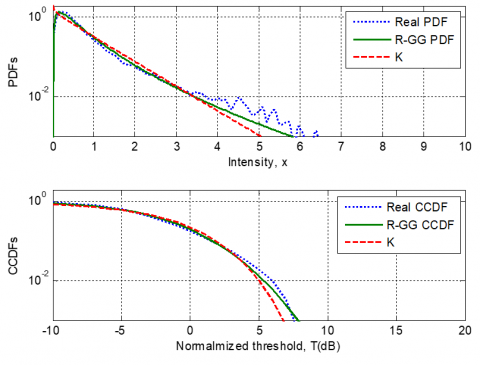Figure 7. Fitting the R-GG and K-distributions to empirical data with HH polarization, resolution 30m and 3rd range cell

The effect of cell resolutions, antennas polarizations and range cells on the tail fitting of the R-GG and K models to empirical data is investigated. For the case of HH polarization, resolution 3m and 13th range cell, Figure 6 shows fitting the theoretical models to real data. Compared to the K-distribution, it is clear that the R-GG is well approximated to real PDF and CCDF. Another study is also investigated when a scenario of data is recorded for HH polarization, resolution 30m and 3rd range cell. Figure 7 highlights also fitting degradation of the K model against the proposed R-GG model. For VV polarization, resolution 15m and 19th range cell, Figure 8 displays fitting results of theoretical distributions against empirical data. Here, the tail fitting errors are remarkable in this scenario of the data. As mentioned in the introduction section, the problem of modeling of sea clutter is the selection of the appropriate texture component distribution. For instance, the log-normal, inverse gamma and inverse Gaussian models have been proved to fit real data [4, 5, 9]. It is worth noted from the previous works that it is not possible to obtain the universal distribution for real data in all cases. The empirical data scene given in Figure 8 should deviates from the R-GG model. Figure 9 depicts PDF and CCDF curves for the case of VV polarization, resolution 3m and 26th range cell. The curves corresponding to each model are completely overlapped. This means that this scenario of data follows approximately both the K and R-GG distributions.

## 8.png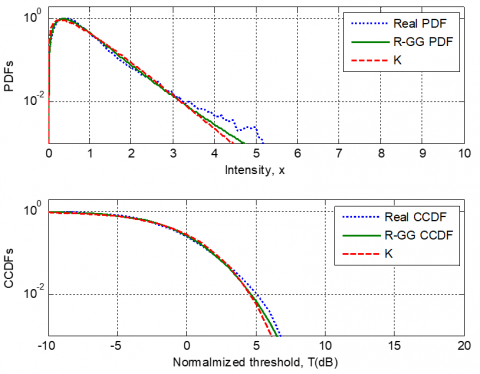Figure 8. Fitting the R-GG and K-distributions to empirical data with VV polarization, resolution 15m and 19th range cell

## 9.pngFigure 9. Fitting the R-GG and K-distributions to empirical data with VV polarization, resolution 3m and 26th range cell

5. Conclusions

In this paper, it was shown that parameters of R-GΓ distributed radar clutter can converge to their real values using some combinations of integer order moments, non-integer order moments and logarithmic moments. Specifically, estimates of underling parameters could be approximated by the application of methods based on HOME, NIOME and [zlog(z)] involving numerical solutions of nonlinear systems. In most cases, this work has confirmed by means of simulated and real data that it is possible to obtain the best fit to real data if the R-GΓ model with [zlog(z)] estimator are taken into account.

References

 Sekine, M., Mao, Y. (1988). Weibull Radar Clutter. Peter Peregrinus Ltd., London, UK. http://dx.doi.org/10.1049/PBRA003E

 Anastassopoulos, V., Lampropoulos, G.A., Drosopoulos, A., Rey, M. (1999). High resolution radar clutter statistics. IEEE Transactions on Aerospace and Electronic Systems, 35(1): 43-60. http://dx.doi.org/10.1109/7.745679

 Bocquet, S. (2015). Parameter estimation for Pareto and K distributed clutter with noise. IET Radar Sonar Navig, 9(1): 104-113. http://dx.doi.org/10.1049/iet-rsn.2014.0148

 Mezache, A., Sahed, M., Soltani, F., Chalabi, I. (2013). A model for non-Rayleigh sea clutter amplitudes using compound inverse Gaussian distribution. In Proceedings of International Radar Conference, Ottawa, Canada, pp. 1-3. http://dx.doi.org/10.1109/RADAR.2013.6585989

 Gouri, A., Mezache, A., Oudira, H., Bentoumi, A., (2016). Mixture of compound-Gaussian distributions for radar sea-clutter modeling. 4th International Conference on Control Engineering & Information Technology (CEIT), Hammamet, Tunisia. http://dx.doi.org/10.1109/CEIT.2016.7929099

 Abraham, D.A., Gelb, J.M., Oldag, A.W. (2010). K-Rayleigh mixture model for sparse active sonar clutter. OCEANS '10 IEEE Conference, Sydney, NSW. http://dx.doi.org/10.1109/OCEANSSYD.2010.5603815

 Weinberg, G.V. (2017). Radar Detection Theory of Sliding Window Processes. CRC Press, New York. http://dx.doi.org/10.1201/9781315154015

 Chong, H., Feng, L., Linrang, Z., Yifei, F., Shuailin, C. (2016). Widening valid estimation range of multilook Pareto shape parameter with closed-form estimators. Electronics Letters, 52(17): 1486-1488. http://dx.doi.org/10.1049/el.2016.1636

 Chalabi, T., Mezache, A. (2019). Estimators of compound Gaussian clutter with log-normal Texture. Taylor & Francis, Remote Sensing Letters, 10(7): 706-716. http://dx.doi.org/10.1080/2150704X.2019.1601275

 Yu, H., Shui, P.L., Huang, Y.T. (2016) Low-order moment-based estimation of shape parameter of CGIG clutter model. Electronics Letters, 52(18): 1561-1563. http://dx.doi.org/10.1049/el.2016.2248

 Abramowitz, M., Stegun, I.A. (1970) Handbook of Mathematical Functions. New York: Dover Publications, Inc. http://dx.doi.org/10.1119/1.1972842

 Ouria, A., Toufigh, M. (2009). Application of Nelder-Mead simplex method for unconfined seepage problems. Applied Mathematical Modelling, 33(9): 3589-3598. https://doi.org/10.1016/j.apm.2008.12.001

 Bakker, R.B., Currie, B. (2019). The McMaster IPIX Radar Sea Clutter Database. http://soma.ece.mcmaster.ca/ipix/, accessed on Oct. 07, 2019.